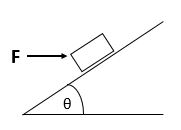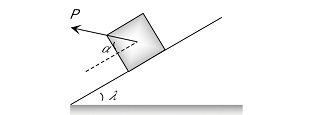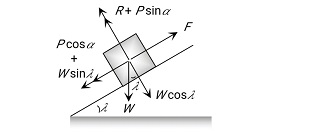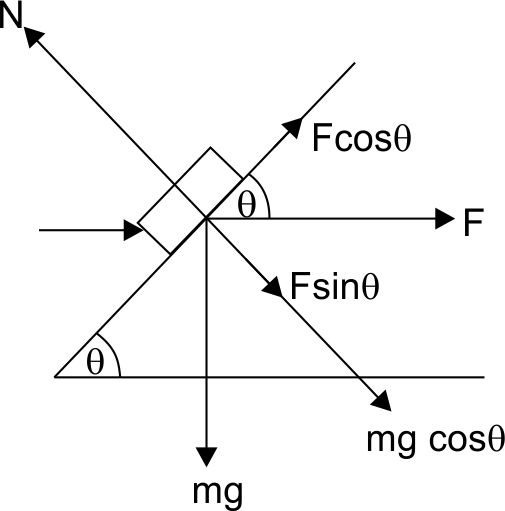#### The fig shows a horizontal forceacting on a block of mass 'M' on an inclined plane. What is the normal reaction of the block?Option 1)Option 2)Option 3)Option 4)As we learnt in

Minimum Force to move a body downward along the surface of Inclined Plane -Use condition of equilibrium.Use- wherein

P = Pulling forceAngle of P with horizontalInclined angle

R = Reaction

W = WeightOption 1)This solution is incorrect.

Option 2)This solution is incorrect.

Option 3)This solution is incorrect.

Option 4)This solution is correct.